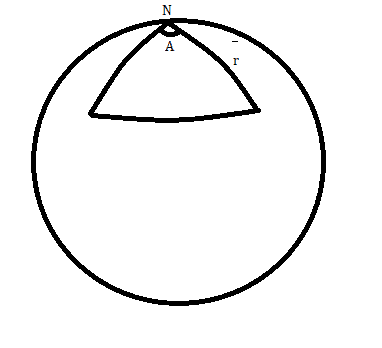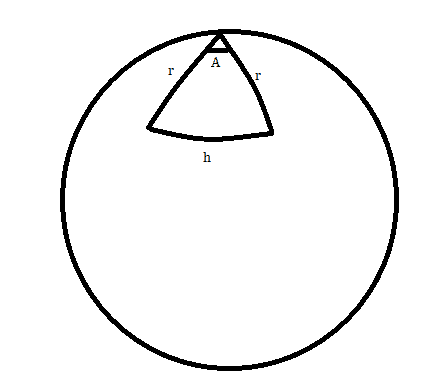# Deriving the area of a spherical triangle from the metric

• I
Gold Member
The metric for 2-sphere is $$ds^2 = dr^2 + R^2sin(r/R)d\theta^2$$

Is there an equation to describe the area of an triangle by using metric.

Note: I know the formulation by using the angles but I am asking for an equation by using only the metric.

jeff davis
Hello,
I think that the area of a triangle in metric would be the same as in imperial. It is just a ratio really. 1/2 B * H
would be the same for metric right?

unless you take the sector and neglect the segment.; Triangle = sector - segment. You could probably derive this in radians easily enough.

jeff

Gold Member
Hello,
I think that the area of a triangle in metric would be the same as in imperial. It is just a ratio really. 1/2 B * H
would be the same for metric right?

unless you take the sector and neglect the segment.; Triangle = sector - segment. You could probably derive this in radians easily enough.

jeff
I did not understand what you are trying to mean.

Gold Member
Well kind of. But I understand the problem ...so I guess there's no need

Staff Emeritus
Homework Helper
Gold Member
Is there an equation to describe the area of an triangle by using metric.
It is unclear what you mean. You obviously need some information about the triangle itself. Which information about the triangle do you want to use? Or are you after a more general integral expression for the area of a part of the sphere?

I think that the area of a triangle in metric would be the same as in imperial.
This is not about units, it is about the metric tensor used in differential geometry as applied to a curved surface.

Gold MemberIt is unclear what you mean. You obviously need some information about the triangle itself
Yes I realized that after some time later. First I thought that from the metric we can define a general area equation for any spherical triangle. However as you mentioned that's possible.. Let's suppose we have a triangle like this. N is the north pole and it makes an angle of A. From the metric I find that the angle can be expressed as

$$Area = \int_0^A \int_0^r Rsin(r/R)drd\theta$$

Last edited:
Staff Emeritus
Homework Helper
Gold Member
Your triangle is not well defined, you need to specify three numbers to completely specify the triangle.

Gold Member
Your triangle is not well defined, you need to specify three numbers to completely specify the triangle.
Well yes sorry the other length is also r. So the bottom length becomes ##ARsin(r/R)dr## (from ##ds = Rsin(r/R)d\theta)##

Actually the area is $$Area = \int_0^rARsin(r/R)dr$$ but this is also equal to $$Area = \int_0^A \int_0^r Rsin(r/R)drd\theta$$

Staff Emeritus
Homework Helper
Gold Member
That is not a triangle. A triangle has geodesics as its sides and the line with the bottom length you have specified is not a geodesic unless ##r## is the distance from the pole to the equator.

Gold Member
That is not a triangle. A triangle has geodesics as its sides and the line with the bottom length you have specified is not a geodesic unless ##r## is the distance from the pole to the equator.
Hmm I know that ##r ∈[0, \pi R/2)##. But that's confusing. I mean$$ds^2 = dr^2 + R^2sin(r/R)^2d\theta^2$$ so for ##h##, ##dr = 0 (?)##.

I see you are meaning that if ##r\ne \pi R/2## then ##dr\ne0##

If we know the value of the A is it possible to find the h from sherical trigonometry (without knowing the value of ##r##)? I guess metric is useless to find the ##h## then

Staff Emeritus
Homework Helper
Gold Member
Unless you know three parameters describing the triangle, it is impossible to find the others. This is true also in Euclidean space. If you do not know r, it is impossible to find h just based on the angle.

Gold Member
Hmm I see. I ll try to look something else for solution. Thanks then

Gold Member
line with the bottom length you have specified is not a geodesic unless rrr is the distance from the pole to the equator.
I ma kind of having trouble to understand this. At post 11 $$h = ARsin(r/R)$$ ? I mean it has to be that way..

Staff Emeritus
Homework Helper
Gold Member
I ma kind of having trouble to understand this. At post 11 $$h = ARsin(r/R)$$ ? I mean it has to be that way..
Again, this is not a triangle. It is a circle sector.

Gold Member
Again, this is not a triangle. It is a circle sector.
Okay.. umm then how can I find the area of that thing. What should I do ? Is it better maybe to use spherical coordinates ?

Staff Emeritus
Homework Helper
Gold Member
Of the circle sector or the triangle?

Gold Member
Of the circle sector or the triangle?
Triangle

Gold Member
The metric for 2-sphere is $$ds^2 = dr^2 + R^2sin(r/R)d\theta^2$$

Here, by ##r## do you mean ##R \theta##? Then your metric would be the more familiar form.
$$ds^2 = R^2 ( d^2 \theta + \sin^2(\theta ) d^2 \phi )$$

And ##\theta## would have range 0 to ## \pi## and ##\phi## would have range 0 to ##2 \pi##. Then it all becomes very familiar surface-of-a-sphere type geometry. Is that it?

•Arman777
Gold Member
Well that could have helped I guess, yes.
But I used the spherical trigonometry to find the area of the triangle.. But the metric you wrote also seems well for my problem as well. Sadly I already handed out the paper...